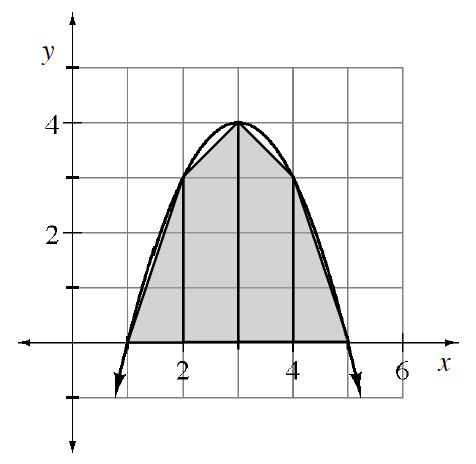Home > APCALC > Chapter 1 > Lesson 1.2.1 > Problem1-25

1-25.

The parabola $y = -(x - 3)^2 + 4$ is graphed at right. Four trapezoids of equal width are inscribed for $1 ≤ x ≤ 5$

1. Use the combined area of these trapezoids to approximate the area under the parabola for $1 ≤ x ≤ 5$.

$\text{Area of a trapezoid}= \frac{1}{2}\left(b_1+b_2\right)h$

It might seem silly now, but the area of a triangle can also be found with formula above. After all, a triangle is a trapezoid with only one base: $b_2 = 0$. Similarly, a rectangle is a trapezoid with two equal bases: $b_1 = b_2$.

2. Is this area greater or less than the true area under the parabola? Explain.

You can visualize the answer to this question. Do you see empty area between the parabola and the trapezoids, or do the trapezoids fill up more area than the parabola fills?